# What is the correct format to export dates with html5 to excel?

These are my dates in datatables (dd/mm/yyyy).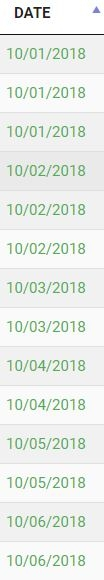Then I export these dates with the excel button.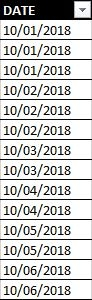As you can see the dates are in the left side and excel treats them as a general format.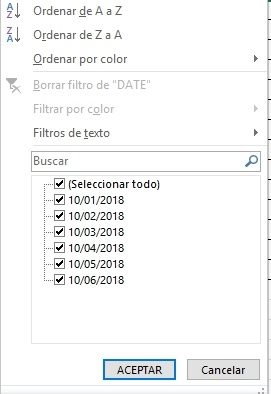Then if I double click each row excel treats theam as date format (right side of the column)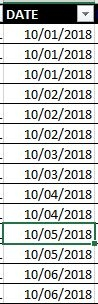and now I can use the date filters correctly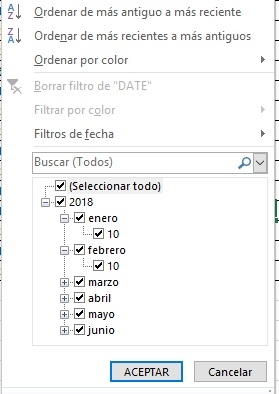I tried dd/mm/yyyy and yyyy-mm-dd formats and it does not work I always need to double click on each row.
The only thing I need is not to use the double click again each row.

Hi @jfabian ,

These two threads here and here should help, it looks like people got it working in the past,

Cheers,

Colin

• Hi @colin neither of the two worked. I don't mind using ISO8601 dates
but it does not automatically detect them. It seems that @ajhulsebos or @mrmccrac are the closest but I can't get it to work.

Hi @jfabian ,

Would you be able to link to the page with the problem, or create a test case that reproduces the problem? That would help to understand the issue,

Cheers,

Colin

• @colin @allan I find the answer !!! (after a week).

First we have this line.

``````<cellXfs count="67">
``````

We change it to this

``````<cellXfs count="68">
``````

Then we have this second line.

``````   '<xf numFmtId="2" fontId="0" fillId="0" borderId="0" applyFont="1" applyFill="1" applyBorder="1" xfId="0" applyNumberFormat="1"/>'+
'</cellXfs>'+
``````

We add the line beetween. The number 14 is really important because is the date format.

``````  '<xf numFmtId="2" fontId="0" fillId="0" borderId="0" applyFont="1" applyFill="1" applyBorder="1" xfId="0" applyNumberFormat="1"/>'+
'<xf numFmtId="14" fontId="0" fillId="0" borderId="0" applyFont="1" applyFill="1" applyBorder="1" xfId="0" applyNumberFormat="1"/>'+
'</cellXfs>'+
``````

It also needs to change _excelSpecials.

``````var _excelSpecials = [
{ match: /^\-?\d+\.\d%\$/,       style: 60, fmt: function (d) { return d/100; } }, // Precent with d.p.
{ match: /^\-?\d+\.?\d*%\$/,     style: 56, fmt: function (d) { return d/100; } }, // Percent
{ match: /^\-?\\$[\d,]+.?\d*\$/,  style: 57 }, // Dollars
{ match: /^\-?£[\d,]+.?\d*\$/,   style: 58 }, // Pounds
{ match: /^\-?€[\d,]+.?\d*\$/,   style: 59 }, // Euros
{ match: /^\-?\d+\$/,            style: 65 }, // Numbers without thousand separators
{ match: /^\-?\d+\.\d{2}\$/,     style: 66 }, // Numbers 2 d.p. without thousands separators
{ match: /^\([\d,]+\)\$/,        style: 61, fmt: function (d) { return -1 * d.replace(/[\(\)]/g, ''); } },  // Negative numbers indicated by brackets
{ match: /^\([\d,]+\.\d{2}\)\$/, style: 62, fmt: function (d) { return -1 * d.replace(/[\(\)]/g, ''); } },  // Negative numbers indicated by brackets - 2d.p.
{ match: /^\-?[\d,]+\$/,         style: 63 }, // Numbers with thousand separators
{ match: /^\-?[\d,]+\.\d{2}\$/,  style: 64 }  // Numbers with 2 d.p. and thousands separators
];
``````

We add at the end one line don't forget the comma. If the regex inside match is wrong or there is no match with the other regex excel will alert that the file is damage. d inside Date.parse(d) must be ISOdate(example: "2018-07-06 "). So at the end the match returns a number with a date format . If we give the date "2018-07-06" it will give to excel the number "43287" with date format so excel will show "06/07/2018".

``````var _excelSpecials = [
{ match: /^\-?\d+\.\d%\$/,       style: 60, fmt: function (d) { return d/100; } }, // Precent with d.p.
{ match: /^\-?\d+\.?\d*%\$/,     style: 56, fmt: function (d) { return d/100; } }, // Percent
{ match: /^\-?\\$[\d,]+.?\d*\$/,  style: 57 }, // Dollars
{ match: /^\-?£[\d,]+.?\d*\$/,   style: 58 }, // Pounds
{ match: /^\-?€[\d,]+.?\d*\$/,   style: 59 }, // Euros
{ match: /^\-?\d+\$/,            style: 65 }, // Numbers without thousand separators
{ match: /^\-?\d+\.\d{2}\$/,     style: 66 }, // Numbers 2 d.p. without thousands separators
{ match: /^\([\d,]+\)\$/,        style: 61, fmt: function (d) { return -1 * d.replace(/[\(\)]/g, ''); } },  // Negative numbers indicated by brackets
{ match: /^\([\d,]+\.\d{2}\)\$/, style: 62, fmt: function (d) { return -1 * d.replace(/[\(\)]/g, ''); } },  // Negative numbers indicated by brackets - 2d.p.
{ match: /^\-?[\d,]+\$/,         style: 63 }, // Numbers with thousand separators
{ match: /^\-?[\d,]+\.\d{2}\$/,  style: 64 },  // Numbers with 2 d.p. and thousands separators
{ match: /^[\d]{4}\-[\d]{2}\-[\d]{2}\$/, style: 67, fmt: function (d) {return Math.round(25569 + (Date.parse(d) / (86400 * 1000)));}}//Date yyyy-mm-dd
];
``````
• If anyone wants to change the format input you only need to change the regex expression and give Date.parse an ISOdate or if you want to change the format output you need to change the number inside numFmtId.

Hi @jfabian ,

Thanks for reporting back, that's good information. Glad you got it working!!

Cheers,

Colin

• if someone want to change the input format to "dd/mm/yyyy" use this line.

``````{ match: /^[\d]{2}\/[\d]{2}\/[\d]{4}\$/, style: 67, fmt: function (d) {return Math.round(25569 + (Date.parse(d.substring(4,8)+"-"+d.substring(2, 4)+"-"+d.substring(0, 2)) / (86400 * 1000)));}}//Date dd/mm/yyyy
``````
• @jfabian working like a charm! I have one question, since i'm not familiarized with regex, is it too dificult to obtain the same but for dd/mm/yyyy hh:mm?

• @Alvii for that format you must give excel a float and change the value 14 for the correct format (I don´t know which number is but it exist in excel).

The regex expresion should be something like this:
`/^[\d]{2}\/[\d]{2}\/[\d]{4}[\s]{1}[\d]{2}\:[\d]{2}\$/`

you need to change the function because Date.parse() won´t give you the float you need.

• edited July 2018 Answer ✓

Thanks for all @jfabian! I got it working. For anyone with the same problem, the numFmtId is 22, and the match line should be like this (in my case):

``````{ match: /^[\d]{2}\/[\d]{2}\/[\d]{4}[\s]{1}[\d]{2}\:[\d]{2}\$/, style: 68, fmt: function (d) { return Math.round(25569 + (Date.parse(d.substring(4, 8) + "-" + d.substring(2, 4) + "-" + d.substring(0, 2)) / (86400 * 1000))) + d.substring(8, 10) / 24 + d.substring(10, 12) / 1440; } },//Date dd/mm/yyyy hh:mm
``````
• @jfabian, sorry to bother you again, but i encountered a problem and maybe you can help me. I'm using your code for "dd/mm/yyyy" dates, and it works well except for dates that start with a 0, for example, 02/08/2018. Are you having the same problem?

@jfabian, i found a solution! By following what @ajhulsebos said in another post, i had to change this:

``````if ( row[i].match && ! row[i].match(/^0\d+/) && row[i].match( special.match ) ) {
``````

for this:

``````if (row[i].match && (!row[i].match(/^0\d+/) || special.style >= 67) && row[i].match(special.match)) {
``````

It's all working perfectly now, thank you both!

• Thanks @Alvii I didn´t see that one. Sorry for the late response.

• Using latest versions of "DT 1.10.20" and "button.html5 1.6.0", I can't get Excel to properly detect date...
I'm using minified datatable file and I don't know where to insert code proposed by @jfabian, so probaly inserted the code at erong place...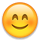I've tried ISO8601 export, but than I need to double click each cell...
1. I would like to export one column that holds "mm.dd.yyyy" and tell Excel to treat it like Date (or Custom format), for proper sorting...
2. Another column I'm exporting is in "hh:mm:ss" format, which also needs to be formated as Time *or Custom) in Excel.
Numbers are trated OK in my case, didn't have to change anything.
I'll continue to try on my own, if anyone has time to comment it would be most welcome!

• Got some progress editing "datatables.min.js"...
Now, I can see changed "style.xml", but Excel complains about damaged data, it does automatic repair and "dates" can be sorted properly (except the ones starting with "0").
I can't find where to put `|| special.style >= 67`, because in "datatables.min.js" everything is named differently.

Hi @fermevc ,

We're happy to take a look, but as per the forum rules, please link to a test case - a test case that replicates the issue will ensure you'll get a quick and accurate response. Information on how to create a test case (if you aren't able to link to the page you are working on) is available here.

Cheers,

Colin

• @colin,
Thnaks for reply, I'll try to gather all info and create a sepparate post with test case...

Hi all,

thanks for this great thread - helped me a lot with the date formatting.
Unfortunately I cannot get my time columns to be exported as actual time values into excel.

In my DB I have 2 datetime columns (start and leave times). As a third column I have a break column in minutes and I calculate a working time (leave-start-break) as well as a presence time (leave-start).

So in total it's 5 columns which are all displayed as h:m. If I export to excel I only get strings but not time values which I can use for calculation.

What I did so far in the excelhtml5.js is to change

``````<cellXfs count="68">
``````

to

``````<cellXfs count="69">
``````

``````<xf numFmtId="46" ...
``````

(also tried 18, 19, 20).

``````{ match: /^[\d]{2}\:[\d]{2}\$/, style: 68 }
``````

The fields setup looks like:

``````        Field::inst( 'start' )
->getFormatter( function ( \$val, \$data, \$opts ) {
return date( 'H:i', strtotime( \$val ) );
}),
Field::inst( 'leave' )
->getFormatter( function ( \$val, \$data, \$opts ) {
return date( 'H:i', strtotime( \$val ) );
}),
Field::inst( 'SEC_TO_TIME(break)' , 'break' )
->getFormatter( function ( \$val, \$data, \$opts ) {
return date( 'H:i', strtotime( \$val ) );
}),
Field::inst( 'timediff(leave, start)', 'presence' )
->getFormatter( function ( \$val, \$data, \$opts ) {
return date( 'H:i', strtotime( \$val ) );
}),
Field::inst( 'timediff(timediff(leave, start), break)', 'working_time' )
->getFormatter( function ( \$val, \$data, \$opts ) {
return date( 'H:i', strtotime( \$val ) );
}),
``````

So either I get a) strings, b) 00:00 values only or c) completely wrong dates like 10.05.2046 00:00:00

Can someonle help me to set this up correctly?

Thank you,
Caspian

We're happy to take a look, but as per the forum rules, please link to a test case - a test case that replicates the issue will ensure you'll get a quick and accurate response. Information on how to create a test case (if you aren't able to link to the page you are working on) is available here.

Cheers,

Colin

Hi Colin,

sorry have overlooked it. I prepared a page here: https://www.lancii.de/zeit/temp.php

Sorry, I misunderstood the problem. This thread here discusses various ways to export in date and time formats - the conclusion is that we don't currently provide that ability but the thread does suggest some tricks. Hope it helps.

• There was a pull request recently for ISO8601 export support, which I have merged in. It isn't in a release version yet, but is available in the nightly. That's only ISO8601 though. You'd need to make a similar code modification if you wanted to support any other format.

Allan

edited November 2019

Thank you, I found these pages but it all is about dates and not times.

I'm trying to figure out how to convert the string into a time but could not get it working yet. So far I managed to get the hours but not the minutes.

``````{ match: /^[\d]{2}\:[\d]{2}\$/, style: 68, fmt: function (d) {return d.substr(0,2)/24;}} //Time hh:mm

``````
Ok, got it (I swear I tried that also yesterday but it didn't work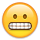)
``````{ match: /^[\d]{2}\:[\d]{2}\$/, style: 68, fmt: function (d) {return d.substr(0,2)/24 + d.substr(2,2)/1440;}} //Time hh:mm